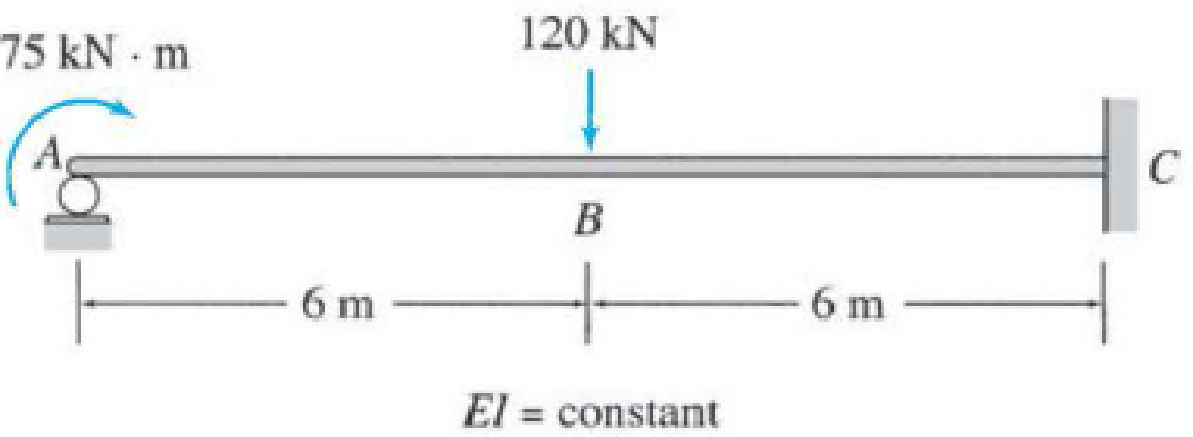# 13.5 through 13.8 Determine the reactions and draw the shear and bending moment diagrams for the beams shown in Figs. P13.1–P13.4 by using the method of consistent deformations. Select the reaction moment at the fixed support to be the redundant. FIG. P13.3, P13.7

#### Solutions

Chapter
Section
Chapter 13, Problem 7P
Textbook Problem
117 views

## 13.5 through 13.8 Determine the reactions and draw the shear and bending moment diagrams for the beams shown in Figs. P13.1–P13.4 by using the method of consistent deformations. Select the reaction moment at the fixed support to be the redundant.FIG. P13.3, P13.7To determine

Find the reactions and sketch the shear and bending moment diagrams for the given beam using method of consistent deformation.

### Explanation of Solution

Given information:

Apply the sign conventions for calculating reactions, forces and moments using the three equations of equilibrium as shown below.

• For summation of forces along x-direction is equal to zero (Fx=0), consider the forces acting towards right side as positive (+) and the forces acting towards left side as negative ().
• For summation of forces along y-direction is equal to zero (Fy=0), consider the upward force as positive (+) and the downward force as negative ().
• For summation of moment about a point is equal to zero (Matapoint=0), consider the clockwise moment as negative and the counter clockwise moment as positive.

Calculation:

Show the free body diagram of the beam as shown in Figure 1.

Refer Figure 1.

Consider the horizontal and vertical reaction at C are denoted by Cx and Cy.

Consider the moment at C is denoted by MC.

Consider the vertical reaction at C is denoted by Cy.

The reactions acting in the beam is 4.

The number of Equilibrium reaction is 3.

The degree of indeterminacy of the beam is 1.

Take the moment at C as the redundant.

Modify the Figure 1 as shown in Figure 2.

Refer Figure 2.

The slope at C due to the external loading is denoted by θCO.

The flexibility coefficient representing the slope at C due to unit value of redundant MC is fCC.

Slope of the Primary Beam:

Show the expression for the slope at the hinge support of a simply supported beam due to the point load P as follows:

θ=Pb6lEI(l2b2) (1)

Here, l is the length of the beam, b is the distance of the load from the roller support, E is the modulus of Elasticity, and I is the moment of inertia.

Calculate the slope of the primary beam due to the action of 60kN load alone as follows:

Substitute 6m for b, 12m for l, and 120kN for P in Equation (1).

θ1=120×66×12EI(12262)=1080kNm2EI

Show the expression for the slope at the hinge support of a simply supported beam due to the unit moment M as follows:

θ=Ml6EI (2)

Here, l is the length of the beam, M is the moment acting at the hinge support, E is the modulus of Elasticity, and I is the moment of inertia.

Substitute 75kNm for M and 12m for l in Equation (2).

θ2=75×126EIθ2=150kNm2EI

Calculate the slope of the primary beam as follows:

θCO=θ1+θ2θCO=1080kNm2EI150kNm2EIθCO=1230kNm2EI (3)

Calculate the value of fCC as follows:

Substitute 1kNm for M and 12m for l in Equation (2).

fCC=1×123EIfCC=4kNm/kNmEI (4)

Show the compatibility Equation as follows:

θCO+fCCMC=0 (5)

Modify Equation (5) using Equation (3) and (4)

### Still sussing out bartleby?

Check out a sample textbook solution.

See a sample solution

#### The Solution to Your Study Problems

Bartleby provides explanations to thousands of textbook problems written by our experts, many with advanced degrees!

Get Started

Find more solutions based on key concepts
In Chapter 11 , we explained the concept of windchill factors. The old windchill values were determined empiric...

Engineering Fundamentals: An Introduction to Engineering (MindTap Course List)

Explain how an operating system uses encryption.

Enhanced Discovering Computers 2017 (Shelly Cashman Series) (MindTap Course List)

What encryption standard is currently recommended by NIST?

Principles of Information Security (MindTap Course List)

5. Identify the main components of an information system. What is a mission-critical system?

Systems Analysis and Design (Shelly Cashman Series) (MindTap Course List)

If your motherboard supports ECC DDR3 memory, can you substitute non-ECC DDR3 memory?

A+ Guide to Hardware (Standalone Book) (MindTap Course List)

What are the advantages of the inverter-type welding power supply?

Welding: Principles and Applications (MindTap Course List)